# 基于VAR模型经济增长、产业结构与环境污染关系的实证研究—以云南省为例An Empirical Study on the Relationship between Economic Growth, Industrial Structure and Environmental Pollution Based on VAR Model—Take Yunnan Province as an Example

DOI: 10.12677/JLCE.2018.73014, PDF, HTML, XML, 下载: 559  浏览: 1,617

Abstract: This paper selects various indicators of Yunnan province’s per capita GDP, the proportion of tertiary industry in GDP and the assessment of environmental pollution degree from 1997 to 2016, and constructs VAR models related to economic growth, industrial structure and environmental pollution. Conclusion: 1) There is a co-integration relationship between economic growth, industrial structure and various indicators measuring the degree of environmental pollution. 2) Whether the Kuznets curve of “inverted u-shaped” environment is established depends on the difference between the data of the selected area and the selected environmental pollution indicators. 3) Economic growth plays a dominant role in the fluctuation of environmental pollution measurement indicators. 4) Existing environmental pollution problem households in Yunnan province restrain economic growth, and the environmental problem caused by economic growth is mainly the emission of industrial waste gas.1. 引言

2. 文献综述

3. 云南省经济增长、产业结构和环境污染的现状分析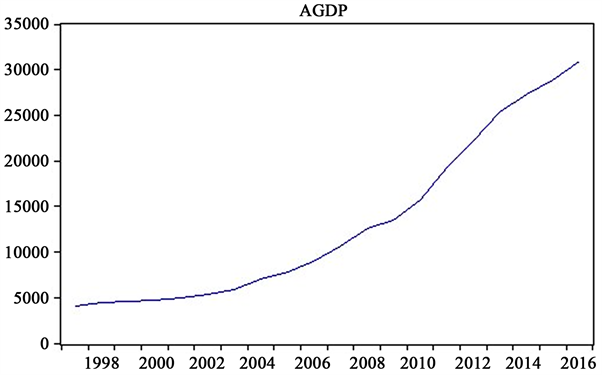Figure 1. Yunnan province per capita GDP timing chart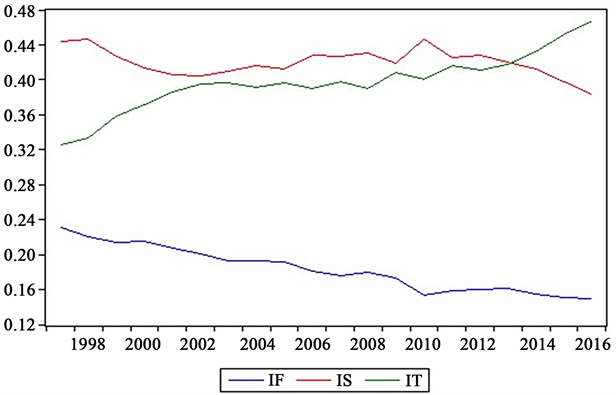注：IF表示第一产业占比、IS表示第二产业占比、IT表示第三产业占比

Figure 2. Yunnan province industrial structure trend chart

4. 模型方法及数据处理

(一) VAR模型

VAR模型即向量自回归模型，在1980年由Sims第一次提出。VAR模型的特点是用将所有当期变量作为因变量，所有变量的若干滞后作为解释变量进行回归。VAR的模型主要用于预测随机干扰项对于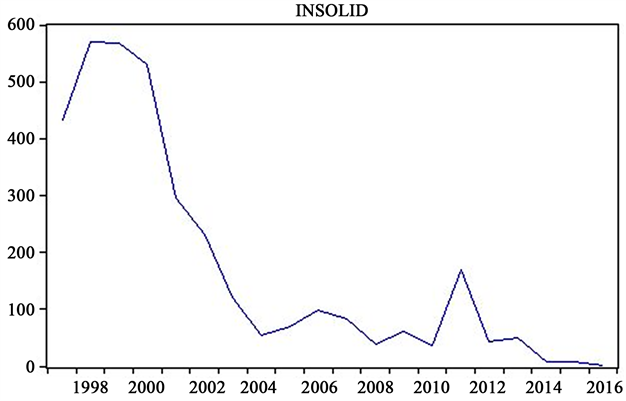Figure 3. Trend chart of total industrial solid waste emission in Yunnan province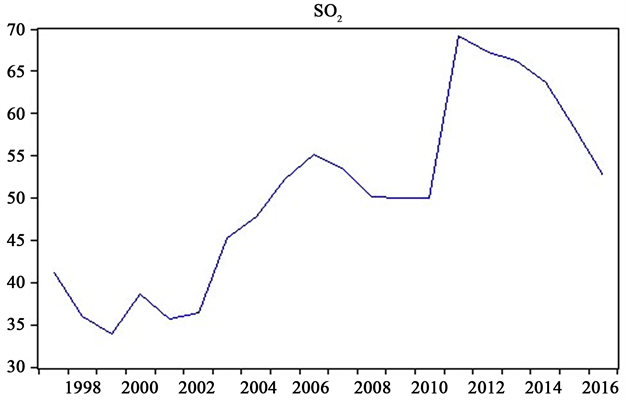Figure 4. Sulfur dioxide emission trend chart of Yunnan province

VAR定义式为 ${Y}_{t}={\left({y}_{1t}\text{\hspace{0.17em}}\text{\hspace{0.17em}}{y}_{2t}\text{\hspace{0.17em}}\text{\hspace{0.17em}}\cdots \text{\hspace{0.17em}}\text{\hspace{0.17em}}{y}_{Nt}\right)}^{\text{T}}$$N×1$ 阶时间序应变量列向量，则p阶VAR模型(记为VAR(p))：

${Y}_{t}={\sum }_{1}^{p}{\Pi }_{i}{Y}_{t-i}+{\mu }_{t}={\Pi }_{1}{Y}_{t-1}+{\Pi }_{2}{Y}_{t-2}+\cdots +{\Pi }_{p}{Y}_{t-p}+{\mu }_{t}$ (1.1)

${\mu }_{t}~IID\left(0,\Omega \right)$

${\mu }_{t}={\left({\mu }_{1t}\text{\hspace{0.17em}}\text{\hspace{0.17em}}{\mu }_{2t}\text{\hspace{0.17em}}\text{\hspace{0.17em}}{\mu }_{3t}\text{\hspace{0.17em}}\text{\hspace{0.17em}}\cdots \text{\hspace{0.17em}}\text{\hspace{0.17em}}{\mu }_{Nt}\right)}^{\text{T}}$

${\mu }_{t}$ 可以同期相关，但通常不与自己的滞后值相关，也不与等式右边的变量相关；

$\Omega$$N×N$ 阶方差协方差矩阵；

P为滞后阶数。

${Y}_{t}={\sum }_{1}^{2}{\Pi }_{i}{Y}_{t-i}+{\mu }_{t}={\Pi }_{1}{Y}_{t-1}+{\Pi }_{2}{Y}_{t-2}+{\mu }_{t}$

$\left(\begin{array}{c}{y}_{t}\\ {x}_{t}\end{array}\right)=\left(\begin{array}{cc}{\pi }_{111}& {\pi }_{112}\\ {\pi }_{121}& {\pi }_{122}\end{array}\right)\left(\begin{array}{c}{y}_{t-1}\\ {x}_{t-1}\end{array}\right)+\left(\begin{array}{cc}{\pi }_{211}& {\pi }_{212}\\ {\pi }_{221}& {\pi }_{222}\end{array}\right)\left(\begin{array}{c}{y}_{t-2}\\ {x}_{t-2}\end{array}\right)+\left(\begin{array}{c}{\mu }_{1t}\\ {\mu }_{2t}\end{array}\right)$

$\left\{\begin{array}{l}{y}_{t}={\pi }_{111}{y}_{t-1}+{\pi }_{112}{x}_{t-1}+{\pi }_{211}{y}_{t-2}+{\pi }_{212}{x}_{t-2}+{\mu }_{1t}\\ {x}_{t}={\pi }_{121}{y}_{t-1}+{\pi }_{122}{x}_{t-1}+{\pi }_{221}{y}_{t-2}+{\pi }_{222}{x}_{t-2}+{\mu }_{2t}\end{array}$

(二) 滞后阶数选择

VAR模型中最佳滞后阶数即p值越小，残差有可能存在自相关的问题，所带来的后果是参数估计不具有一致性。增加p值可消除残差中自相关问题。然而p值不能太大，p值太大，待估参数变多，所带来不利影响是自由度严重降低，直接影响参数估计的有效性。在这里介绍常用的两种选择p值的方法  。

1) 用SC和AIC准则确定最佳滞后期数，即p值。P值的选取方法为，在p值增大过程中同时使AIC和SC的值最小。

2) 用LR(似然比统计量)选择最佳的p值，其中 $\text{LR}=-2\left[\mathrm{ln}\left(p\right)-\mathrm{ln}\left(p+i\right)\right]~{\chi }_{\alpha }^{2}\left(f\right)$

(三) 指标和数据的选取

5. 实证分析

(一) 单位根检验

(二) 确定最佳滞后阶数

$\begin{array}{c}\text{LR}=-2\left[\mathrm{ln}\left(p\right)-\mathrm{ln}\left(p+i\right)\right]\\ =-2×\left[139.207-160.4601\right]=42.5062>{\chi }_{0.05}^{2}\left(16\right)=26.296\end{array}$

$\begin{array}{c}\left[\begin{array}{c}{\text{lnagdp}}_{t}\\ \begin{array}{c}{\text{lnit}}_{t}\\ {\text{lninwater}}_{t}\\ \text{lns}{o}_{2}{}_{t}\end{array}\end{array}\right]=\left[\begin{array}{c}2.0548\\ \begin{array}{c}-1.1488\\ 1.6183\\ 2.1058\end{array}\end{array}\right]+\left[\begin{array}{c}\begin{array}{ccc}\begin{array}{cc}1.001& -0.091\end{array}& -2.045& 0.268\end{array}\\ \begin{array}{c}\begin{array}{ccc}\begin{array}{cc}-0.096& 0.042\end{array}& 0.082& -0.150\end{array}\\ \begin{array}{cc}0.102& \begin{array}{ccc}-2.633& 0.543& -0.053\end{array}\end{array}\\ \begin{array}{ccc}1.339& -1.371& \begin{array}{cc}-0.241& 0.489\end{array}\end{array}\end{array}\end{array}\right]\left[\begin{array}{c}{\text{lnagdp}}_{t-1}\\ \begin{array}{c}{\text{lnit}}_{t-1}\\ {\text{lninwater}}_{t-1}\\ \text{lns}{o}_{2}{}_{t-1}\end{array}\end{array}\right]\\ \text{\hspace{0.17em}}\text{\hspace{0.17em}}+\left[\begin{array}{ccc}\begin{array}{c}0.086\\ 0.128\\ \begin{array}{c}0.456\\ -1.075\end{array}\end{array}& \begin{array}{c}0.306\\ 0.574\\ \begin{array}{c}2.029\\ 1.284\end{array}\end{array}& \begin{array}{c}\begin{array}{cc}-0.072& -0.110\end{array}\\ \begin{array}{cc}0.012& -0.012\end{array}\\ \begin{array}{cc}\begin{array}{c}0.052\\ 0.236\end{array}& \begin{array}{c}-0.733\\ -0.551\end{array}\end{array}\end{array}\end{array}\right]\left[\begin{array}{c}{\text{lnagdp}}_{t-2}\\ {\text{lnit}}_{t-2}\\ \begin{array}{c}{\text{lninwater}}_{t-2}\\ \text{lns}{o}_{2}{}_{t-2}\end{array}\end{array}\right]\end{array}$

(三) VAR平稳性检验

(四) Johansen协整检验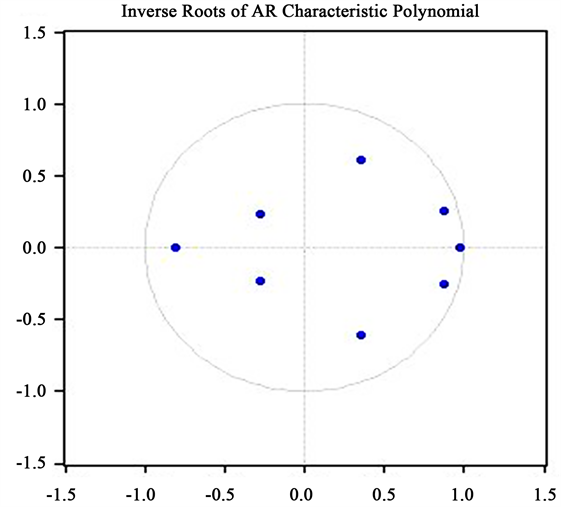Figure 5. Characteristic root profileTable 3. Delayed order decision resultsTable 4. Characteristic root distribution table

No root lies outside the unit circle. VAR satisfies the stability condition.Table 5. Co-integration test results of lnagdp, lnit, lninwater and lnSO2

(五) Granger因果检验

Johansen协整检验显示lnagdp、lnit、lninwater、lnSO2存在协整关系，然而这4个变量之间是否存在相互关系却不得而知，所以要对以上四个时间序列进行Granger因果检验，结果如表6所示。

(六) 基于VAR模型的响应脉冲分析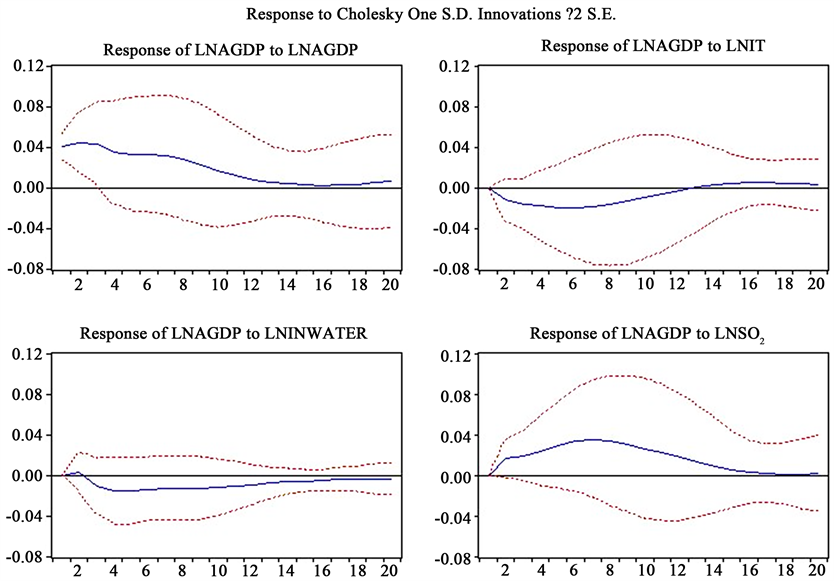Figure 6. Lnagdp impulse response analysis of lnagdp, lnit, lninwater and lnSO2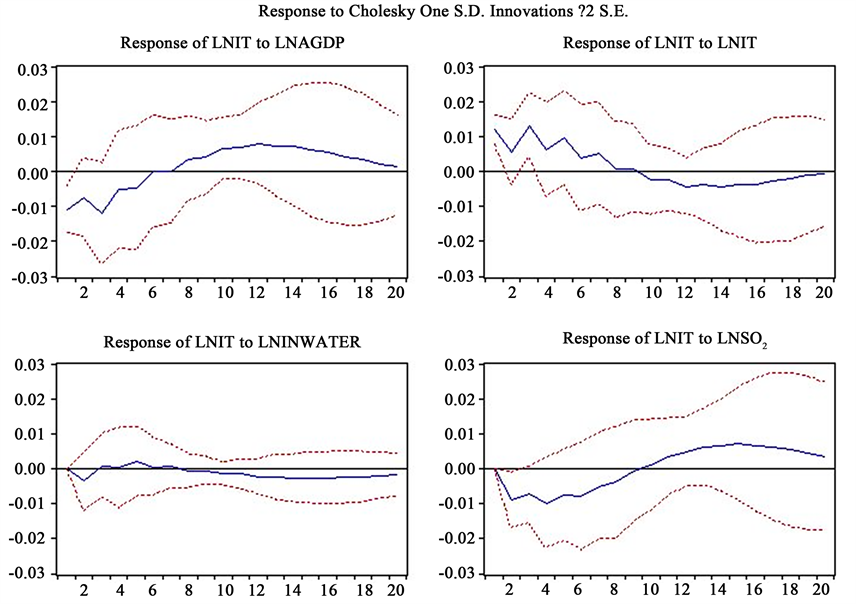Figure 7. lnit impulse response analysis of lnagdp, lnit, lninwater and lnSO2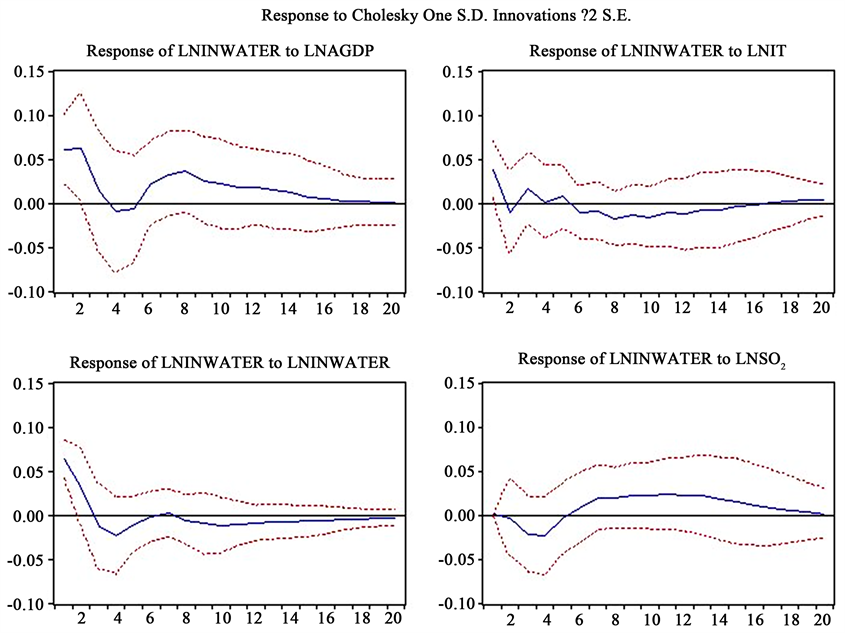Figure 8. Pulse response analysis of lninwater to lnagdp, lnit, lninwater and lnSO2

(七) 基于VAR模型的方差分解分析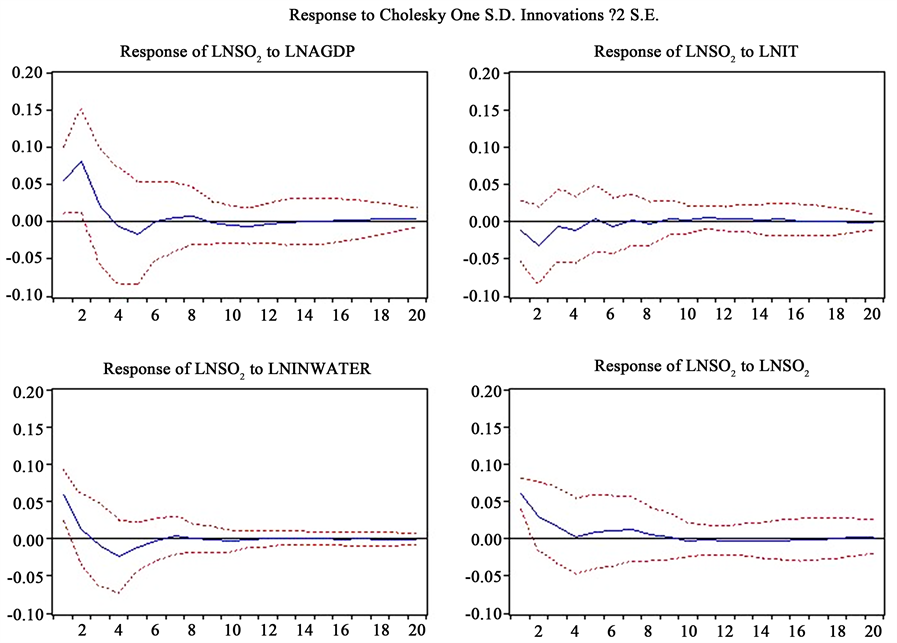Figure 9. Pulse response analysis of lnSO2 to lnagdp, lnit, lninwater and lnSO2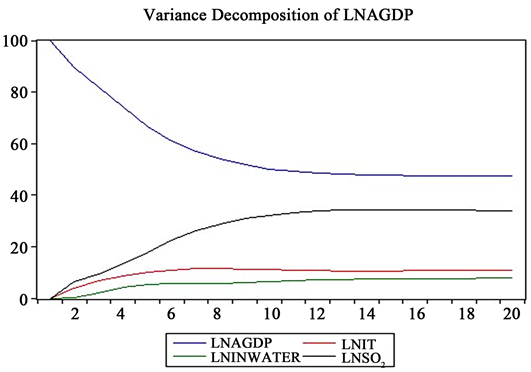Figure 10. Analysis of variance of lnagdp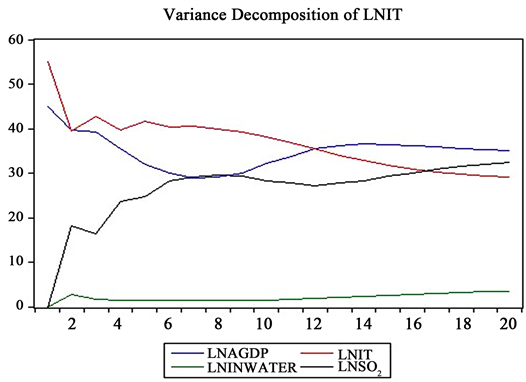Figure 11. Variance decomposition of lnit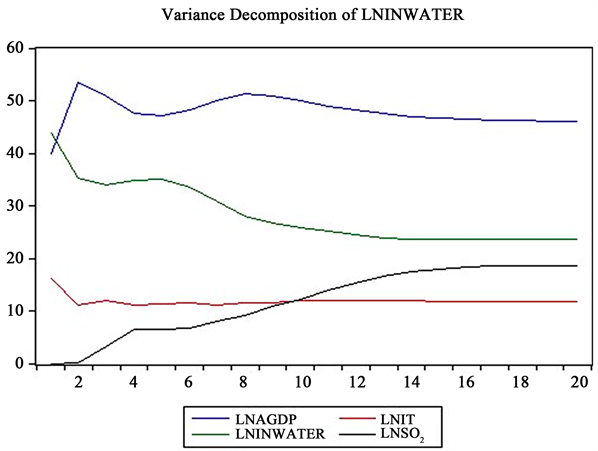Figure 12. Variance decomposition of lninwater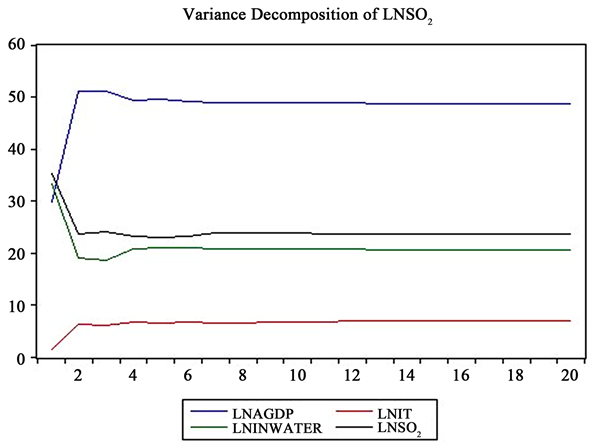Figure 13. Variance decomposition of SO2

6. 结论政策建议

(一) 结论

(二) 政策建议

1) 改变传统的发展观念，加快绿色环保的先进技术的发展  。从长期来看，环境污染会对经济增长带来不利的影响，在环境污染和经济发展之间矛盾日益明显的今天，致力于绿色、低污染的经济发展能够有效的克服这种不利影响。所以贯彻环境治理的理念和加快由粗放向集约型发展到提高资源利用效率、加快可持续发展变得刻不容缓。

2) 加快产业结构转型升级。云南省的第三产业至2013年开始超过第二产业所占比重，第二产业能源消耗大所产生的废物较多，在环境污染占主要解释部分。而相对大力发展第三产业对于环境的污染一般较低。云南省已经初步实现了“三二一”产业结构的转型升级，以2016年为例，三次产业占比分别为14.91%、38.38%、46.71%，第三产业比第二产业多8.33%。当前，云南省以旅游业、金融保险业、房地产业为代表的现代服务业正蓬勃发展，这类行业均属于低污染高附加值的第三产业，对于实现低污染，高产出、实现三次产业结构合理配置起到重要的推动作用。

3) 大力发展先进制造业。在进行产业结构升级转型的过程中也不能忽视第二产业中的贡献，第二产业是所有行业造成环境污染最为严重的部分。目前，云南省工业行业中烟草工业冶金、建材供需矛盾突出，高能耗高污染的粗放集约式的发展已不能适应云南省的经济发展。在“中国制造2025”的环境下，云南省应当大力发展先进的生产技术把握时代契机，升级传统工业，优先发展新能源、新材料等一批新兴产业，为云南省经济可持续发展注入新的动力。

4) 提高信息公开透明度，加大普通群众参与度  。普通民众是环境污染的直接受害者，民众对于处理环境污染的热情较高。民众参与的一个重要前提是信息公开透明。信息不公开，民众无法有效的对政府污染处理工作进行监督。有些地方政府对于环境污染治理的信息不能及时的公开、信息不对称。所以，政府应当提高信息公开透明度，加大普通群众参与度，让民众能够清楚云南省重大环境污染事件，亦或告知群众普通常态数据，让民众参与其中提高环保意识。

5) 强化环保法律的惩罚制度，环境的污染程度越高对于普通民众的伤害也越大，产生这些污染源的企业公司往往注重自身利益的最大化而忽略这些生产的外部性对于普通民众的影响，所以云南省政府应当加大对于企业污染不处理的惩罚力度，让他们认识到不能只关心眼前利益应该立足于长远发展，从而在一个绿色而和谐的环境中共同促进云南省经济可持续发展。

  Grossman, G.M. and Krueger, A.B. (1993) Environmental Impacts of North American Free Trade Agreement, the US Mexico Free Trade Agreement. MIT Press, Cambridge.  Grossman, G.M. and Krueger, A. (1995) Economic Growth and the Environment. Quarterly Journal of Economics, 110, 353-373. https://doi.org/10.2307/2118443  Selden, T. and Song, D. (1994) Envi-ronmental Quality and Development: Is There a Kuznets Curve for Air Pollution Emissions. Journal of Environmental Economics and Management, 27, 147-162. https://doi.org/10.1006/jeem.1994.1031  Galeottim, M. and Lanza, A. (2005) Desperately Seeking Environmental Kuznets. Environmental Modeling & Software, 20, 1379-1388. https://doi.org/10.1016/j.envsoft.2004.09.018  Egli (2003) The En-vironmental Kuznets Curve: A Survey of the Literature. European University Institute.  马树才, 李国柱. 中国经济增长与环境污染关系的Kuznets曲线[J]. 统计研究, 2006(8): 37-40.  张晓. 中国环境政策的总体评价[J]. 中国社会科学, 1999(3): 88-99.  郑长德, 刘帅. 基于空间计量经济学的碳排放与经济增长分析[J]. 人口•资源与环境, 2011, 21(5): 80-86.  包群, 彭水军. 经济增长与环境污染: 基于面板数据的联立方程估计[J]. 世界经济, 2006(11): 48-58.  盖美, 曹桂艳, 田成诗, 等. 辽宁沿海经济带能源消费碳排放与区域经济增长脱钩析[J]. 资源科学, 2014, 36(6): 1267-1277.  徐成龙, 任建兰, 巩灿娟. 产业结构调整对山东省碳排放的影响[J]. 自然资源学, 2014, 29(2): 201-210.  于峰, 齐建国, 田晓林. 经济发展对环境质量影响的实证分析: 基于1999-2004年间各省的面板数据[J]. 中国工业经济, 2006(8): 36-44.  黄少博. 碳排放交易与产业结构和经济增长[D]: [硕士学位论文]. 济南: 山东大学, 2016.  陶长琪, 徐晔, 万建香, 等. 计量经济学[M]. 南京: 南京大学出版社, 2016.  江心英, 赵爽. 江苏省经济增长、产业结构与碳排放关系的实证研究——基于VAR模型和脉冲响应分析[J]. 南京财经大学学报, 2018(2).  李志国, 周德田. 基于VAR模型的经济增长与环境污染关系实证分析——以山东省为例[J]. 企业经济, 2013(8): 11-16.